Next: Relaxation Time Up: Statistical Mechanics Previous: Principle of Equal A

#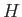-Theorem

Consider a system of weakly-interacting particles. In quantum mechanics, we can write the Hamiltonian for such a system as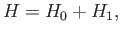(3.4)

where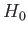is the Hamiltonian for completely non-interacting particles, and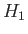a small correction due to the particle interactions. We can define approximate stationary eigenstates of the system using. Thus,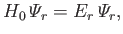(3.5)

where the indexlabels a state of energy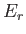and eigenstate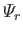. (See Section C.9.) In general, there are many different eigenstates with the same energy--these are called degenerate states. (See Section C.10.)

For example, consider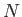non-interacting spinless particles of mass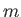confined in a cubic box of dimension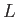. According to standard wave-mechanics, the energy levels of the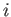th particle are given by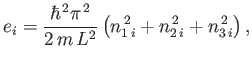(3.6)

where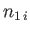,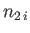, and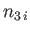are three (positive integer) quantum numbers. (See Section C.10.) The overall energy of the system is the sum of the energies of the individual particles, so that for a general state,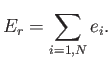(3.7)

The overall state of the system is thus specified by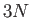quantum numbers (i.e., three quantum numbers per particle). There are clearly very many different arrangements of these quantum numbers that give the same overall energy.

Consider, now, a statistical ensemble of systems made up of weakly-interacting particles. Suppose that this ensemble is initially very far from equilibrium. For instance, the systems in the ensemble might only be distributed over a very small subset of their accessible states. If each system starts off in a particular stationary state (i.e., with a particular set of quantum numbers) then, in the absence of particle interactions, it will remain in that state for ever. Hence, the ensemble will always stay far from equilibrium, and the principle of equal a priori probabilities will never be applicable. In reality, particle interactions cause each system in the ensemble to make transitions between its accessible stationary'' states. This allows the overall state of the ensemble to change in time.

Let us label the accessible states of our system by the index. We can ascribe a time-dependent probability,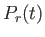, of finding the system in a particular approximate stationary stateat time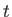. Of course,is proportional to the number of systems in the ensemble in stateat time. In general,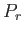is time-dependent because the ensemble is evolving towards an equilibrium state. Let us assume that the probabilities are properly normalized, so that the sum over all accessible states always yields unity: that is,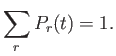(3.8)

Small interactions between particles cause transitions between the approximate stationary states of the system. Thus, there exists some transition probability per unit time,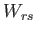, that a system originally in stateends up in state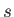as a result of these interactions. Likewise, there exists a probability per unit time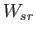that a system in statemakes a transition to state. These transition probabilities are meaningful in quantum mechanics provided that the particle interaction strength is sufficiently small, there is a nearly continuous distribution of accessible energy levels, and we consider time intervals that are not too small. These conditions are easily satisfied for the types of systems usually analyzed via statistical mechanics (e.g., nearly-ideal gases). One important conclusion of quantum mechanics is that the forward and backward transition probabilities between two states are the same, so that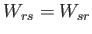(3.9)

for any two statesand. This result follows from the time-reversal symmetry of quantum mechanics. On the microscopic scale of individual particles, all fundamental laws of physics (in particular, classical and quantum mechanics) possess this symmetry. So, if a certain motion of particles satisfies the classical equations of motion (or Schrödinger's equation) then the reversed motion, with all particles starting off from their final positions and then retracing their paths exactly until they reach their initial positions, satisfies these equations just as well.

Suppose that we were to film'' a microscopic process, such as two classical particles approaching one another, colliding, and moving apart. We could then gather an audience together and show them the film. To make things slightly more interesting we could play it either forwards or backwards. Because of the time-reversal symmetry of classical mechanics, the audience would not be able to tell which way the film was running. In both cases, the film would show completely plausible physical events.

We can play the same game for a quantum process. For instance, we could film'' a group of photons impinging on some atoms. Occasionally, one of the atoms will absorb a photon and make a transition to an excited state (i.e., a state with higher than normal energy). We could easily estimate the rate constant for this process by watching the film carefully. If we play the film backwards then it will appear to show excited atoms occasionally emitting a photon, and then decaying back to their unexcited state. If quantum mechanics possesses time-reversal symmetry (which it certainly does) then both films should appear equally plausible. This means that the rate constant for the absorption of a photon to produce an excited state must be the same as the rate constant for the excited state to decay by the emission of a photon. Otherwise, in the backwards film, the excited atoms would appear to emit photons at the wrong rate, and we could then tell that the film was being played backwards. It follows, therefore, that, as a consequence of time-reversal symmetry, the rate constant for any process in quantum mechanics must equal the rate constant for the inverse process.

The probability,, of finding the systems in the ensemble in a particular statechanges with time for two reasons. Firstly, systems in another statecan make transitions to the state. The rate at which this occurs is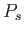, the probability that the systems are in the stateto begin with, multiplied by the rate constant of the transition. Secondly, systems in the statecan make transitions to other states such as. The rate at which this occurs is clearlytimes. We can, thus, write a simple differential equation for the time evolution of: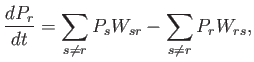(3.10)

or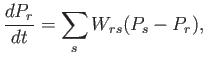(3.11)

where use has been made of the symmetry condition (3.9). The summation is over all accessible states.

Consider now the quantity(from which the-theorem derives its name), which is defined as the average value of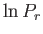taken over all accessible states: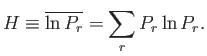(3.12)

This quantity changes as the individual probabilitiesvary in time. Straightforward differentiation of the previous equation yields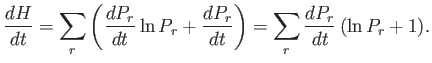(3.13)

According to Equation (3.11), this can be written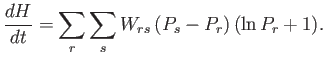(3.14)

We can now interchange the dummy summations indicesandto give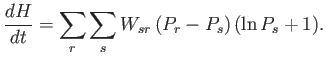(3.15)

Finally, we can write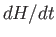in a more symmetric form by adding the previous two equations, and then making use of Equation (3.9):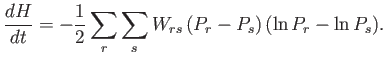(3.16)

Note, however, thatis a monotonically increasing function of. It follows that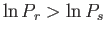whenever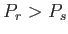, and vice versa. Thus, in general, the right-hand side of the previous equation is the sum of many negative contributions. Hence, we conclude that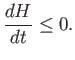(3.17)

The equality sign only holds in the special case where all accessible states are equally probable, so that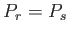for alland. This result is called the-theorem, and was first proved by the unfortunate Professor Boltzmann.

The-theorem tells us that if an isolated system is initially not in equilibrium then it will evolve in time, under the influence of particle interactions, in such a manner that the quantityalways decreases. This process will continue untilreaches its minimum possible value, at which point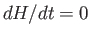, and there is no further evolution of the system. According to Equation (3.16), in this final equilibrium state, the system is equally likely to be found in any one of its accessible states. This is, of course, the situation predicted by the principle of equal a priori probabilities.

The previous argument does not constitute a mathematically rigorous proof that the principle of equal a priori probabilities applies to many-particle systems, because we tacitly made an unwarranted assumption. That is, we assumed that the probability of the system making a transition from some stateto another stateis independent of the past history of the system. In general, this is not the case in physical systems, although there are many situations in which it is a fairly good approximation. Thus, the epistemological status of the principle of equal a priori probabilities is that it is plausible, but remains unproven. As we have already mentioned, the ultimate justification for this principle is empirical--it leads to theoretical predictions that are in accordance with experimental observations.Next: Relaxation Time Up: Statistical Mechanics Previous: Principle of Equal A
Richard Fitzpatrick 2016-01-25